@linux1s1s 2019-02-15T15:11:57.000000Z 字数 7986 阅读 3649

# Android 打包系列-基本打包流程

2016-03 AndroidBuild

## Tools

### aapt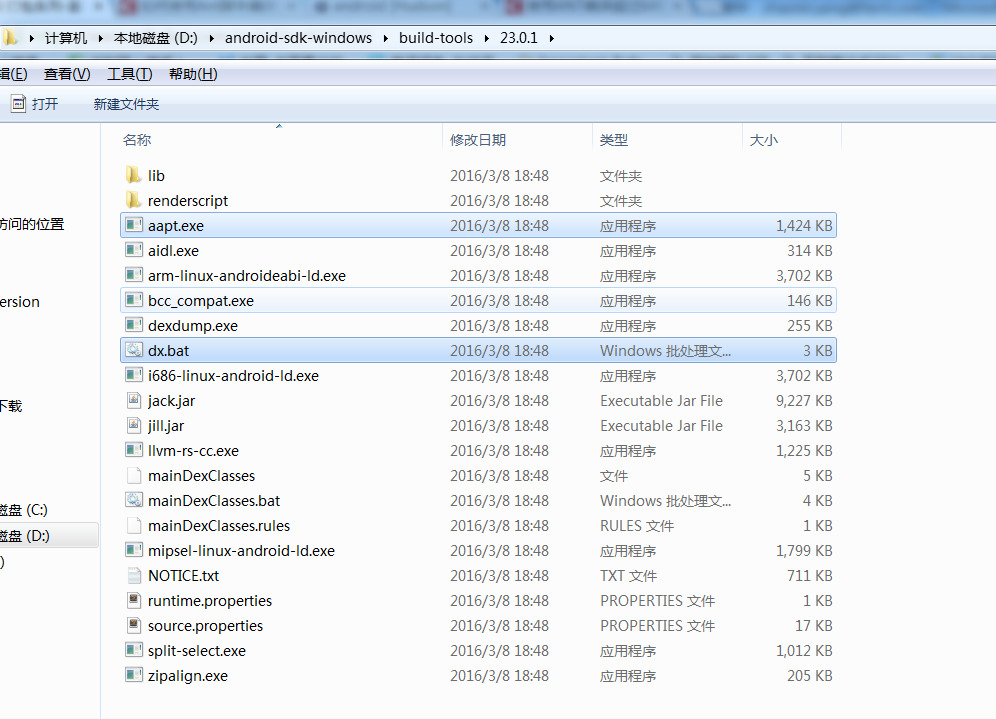- 使用aapt命令编译资源文件

aapt package -f -m -J gen -S res -I D:/android-sdk-windows/platforms/android-16/android.jar -M AndroidManifest.xml 

-J 后面跟着是gen目录，也就是编译之后产生的R类，存放的资源Id
-S 后面跟着是res目录，也就是需要编译的资源目录
-l 后面跟着是系统的库，因为我们在项目资源中会用到系统的一些资源文件，所以这里需要链接一下
-M 后面跟着是工程的清单文件，需要从这个文件中得到应用的包名，然后产生对应的R文件和包名.

• 使用aapt命令生成资源包文件(编码AndroidManifest.xml,resource.arsc等)
aapt package -f -A assets -S res -I D:/android-sdk-windows/platforms/android-17/android.jar -M AndroidManifest.xml -F bin/AntDemo

### javac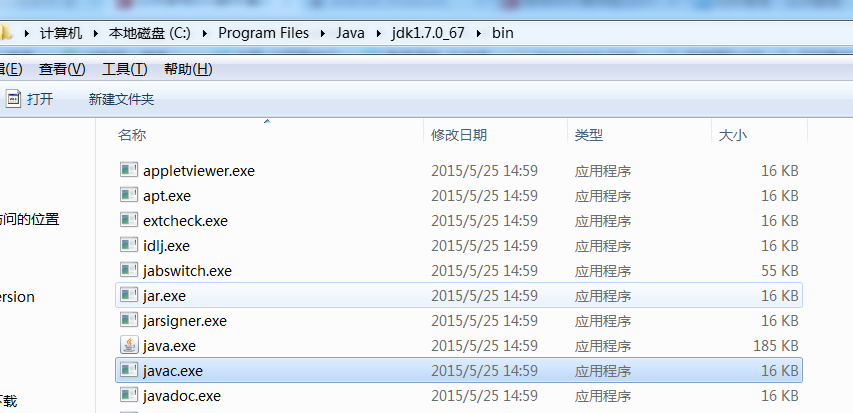javac -target 1.6 -bootclasspath D:/android-sdk-windows/platforms/android-17/android.jar -d bin gen\com\example\antdemo\*.java src\com\example\antdemo\*.java

-target：表示编译之后的class文件运行的环境版本
-bootclasspath：表示编译需要用到的系统库
-d：表示编译之后的class文件存放的目录

### dx

dx --dex --output=G:\Code\Android\Workspace\AntDemo\bin\classes.dex G:\Code\Android\Workspace\AntDemo\bin

dx通配符用法如下，后面系列文章里面会介绍详细用法。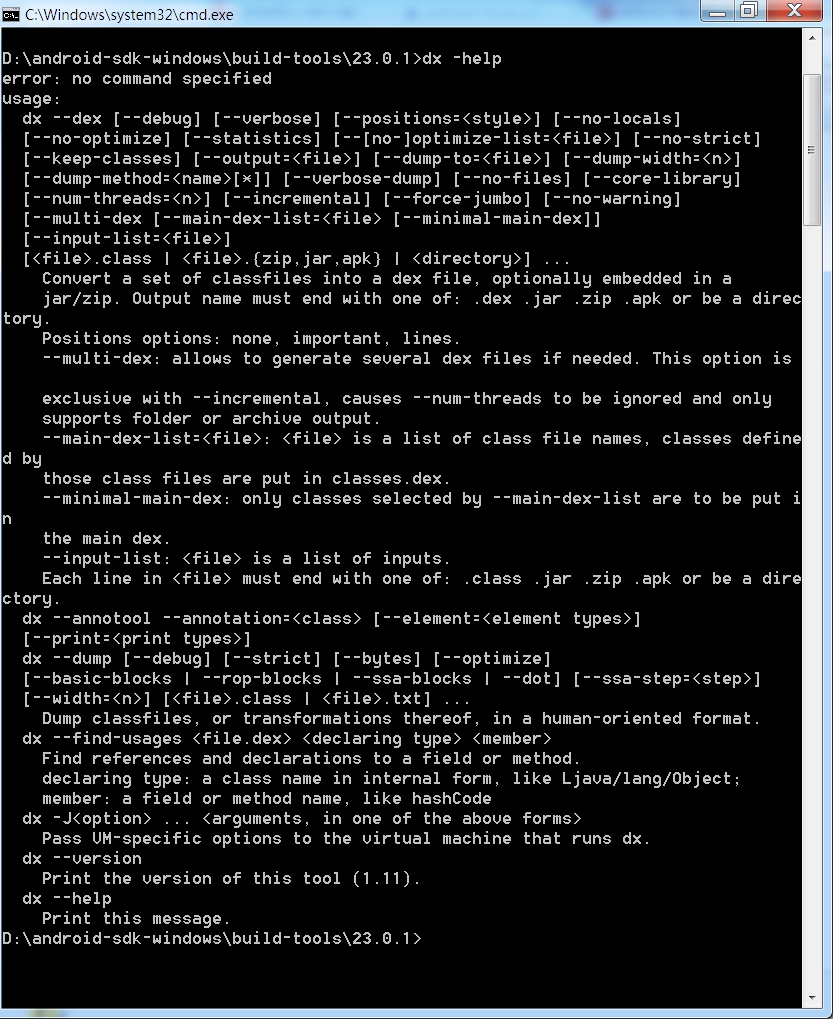### apkbuilder

apkbuilder  ${output.apk.file} -u -z${packagedresource.file} -f  ${dex.file} -rf${source.dir}  -rj  ${libraries.dir} -u 表示 创建一个未签名的apk ${output.apk.file} 表示要输出的apk路径。例如：d:/HelloWorld/bin/my.apk -z${packagedresource.file} 这个为编译好的资源包。 例如：d:/HelloWorld/bin/resources.ap_ -f ${dex.file} 添加的dex文件的路径。例如：d:/HelloWorld/bin/classes.dex -rf${source.dir} 这个为源文件的路径。 例如：d:/HelloWorld/src -rj${libraries.dir} 这个表示引用的库的路径。例如：d:/HelloWorld/libs

### apktool

apktool b xxx xxx_unsigned.apk  

b表示build package，xxx就是刚才你释放出来的文件夹，xxx_unsigned.apk就是重新打包的文件，unsigned的后缀是为了区分这个是未签名的程序，这个时候生成的是一个没有签名的文件，是装不上的，所以下面要增加签名。

apktool d -f xxx.apk xxx  

d表示是decode，-f表示如果目标存在是覆盖。前面那个apk就是你要解包的文件，后面是解出来的文件夹名称。

### keytool

keytool -genkey -alias ant_test1 -keyalg RSA -validity 20000 -keystore my.keystore

### jarsigner

jarsigner -keystore G:\Code\Android\Workspace\AntDemo\build\my.keystore -storepass 123456 -keypass 123456 -signedjar G:\Code\Android\Workspace\AntDemo\bin\AntDemo_signed.apk G:\Code\Android\Workspace\AntDemo\bin\AntDemo_unsigned.apk ant_test

### Zipalign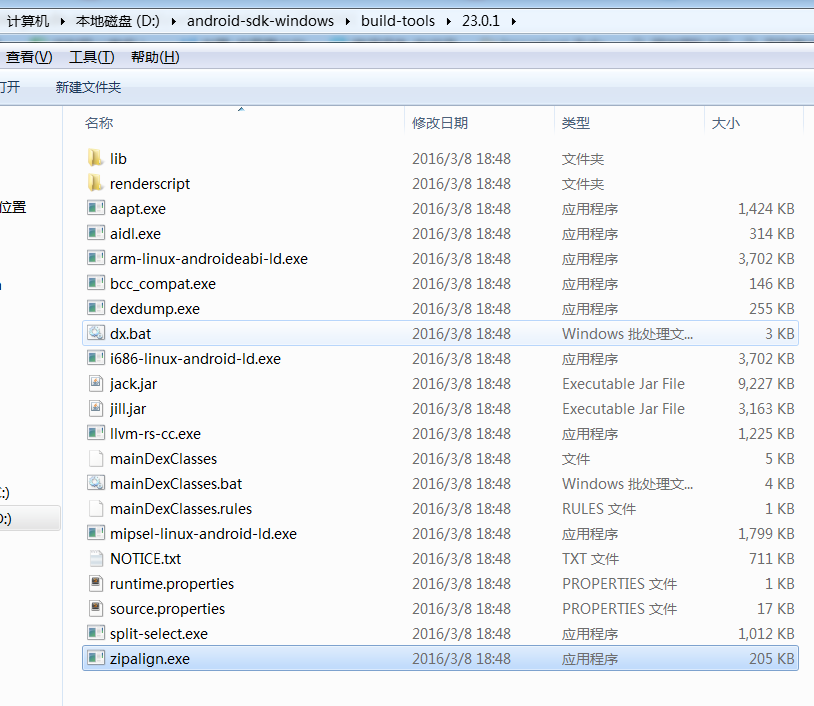zipalign -v 4 source.apk destination.apk

## Flow path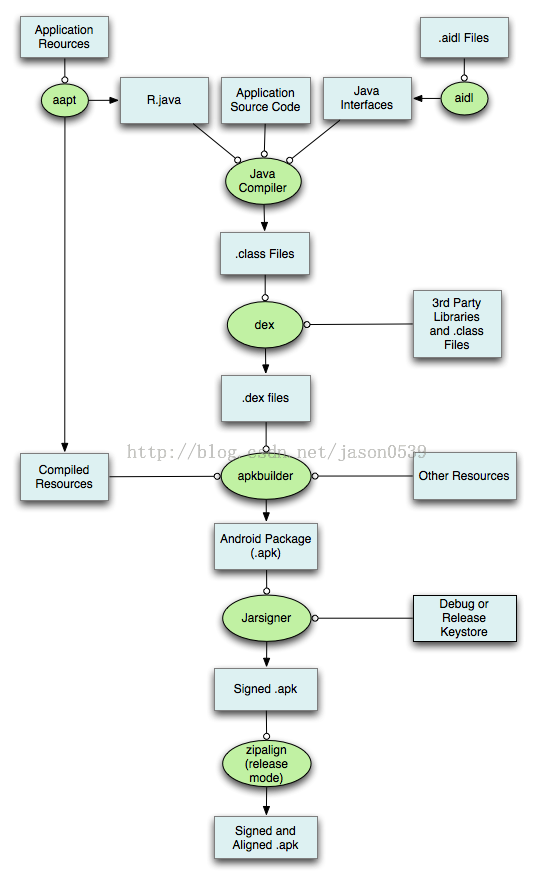## Ant封装打包

<?xml version="1.0" encoding="UTF-8"?><project    name="AntDemo"    default="release" >    <!-- tools dir -->    <property        name="sdk-folder"        value="C:\Users\i\AppData\Local\Android\sdk" />    <property        name="platform-folder"        value="${sdk-folder}\platforms\android-22" /> <property name="platform-tools-folder" value="${sdk-folder}\build-tools\22.0.1" />    <property        name="jdk-folder"        value="C:\Program Files\Java\jdk1.7.0_71" />    <property        name="android-jar"        value="${platform-folder}\android.jar" /> <property name="tools.aapt" value="${platform-tools-folder}\aapt.exe" />    <property        name="tools.javac"        value="${jdk-folder}\bin\javac.exe" /> <property name="tools.dx" value="${platform-tools-folder}\dx.bat" />    <property        name="tools.apkbuilder"        value="${sdk-folder}\tools\apkbuilder.bat" /> <property name="tools.jarsigner" value="${jdk-folder}\bin\jarsigner.exe" />    <!-- project dir -->    <property        name="project-dir"        value="C:\Users\i\Desktop\AntDemo\AntDemo" />    <property        name="res"        value="${project-dir}\res" /> <property name="gen" value="${project-dir}\gen" />    <property        name="src"        value="${project-dir}\src" /> <property name="bin" value="${project-dir}\bin" />    <property        name="assets"        value="${project-dir}\assets" /> <property name="libs" value="${project-dir}\libs" />    <!-- file lists -->    <property        name="manifest"        value="${project-dir}\AndroidManifest.xml" /> <property name="java-file-gen" value="${gen}\com\example\antdemo\*.java" />    <property        name="java-file-src"        value="${src}\com\example\antdemo\*.java" /> <property name="dex-name" value="${bin}\classes.dex" />    <property        name="pakcage-temp-name"        value="${bin}\${ant.project.name}" />    <property        name="unsigned-apk-name"        value="${ant.project.name}_unsigned.apk" /> <property name="unsigned-apk-path" value="${bin}\${unsigned-apk-name}" /> <property name="signed-apk-name" value="${ant.project.name}.apk" />    <property        name="signed-apk-path"        value="${bin}\${signed-apk-name}" />    <property        name="keystore-name"        value="${project-dir}\my.keystore" /> <property name="keystore-alias" value="ant_test" /> <!-- task --> <target name="init" > <echo> Initialize... </echo> <delete dir="${bin}" />        <mkdir dir="${bin}" /> </target> <target name="gen-R" depends="init" > <echo> Generating R.java from the resources...  </echo> <exec executable="${tools.aapt}"            failonerror="true" >            <arg value="package" />            <arg value="-f" />            <arg value="-m" />            <arg value="-J" />            <arg value="${gen}" /> <arg value="-S" /> <arg value="${res}" />            <arg value="-M" />            <arg value="${manifest}" /> <arg value="-I" /> <arg value="${android-jar}" />        </exec>    </target>    <target        name="compile"        depends="gen-R" >        <echo>            Compile...        </echo>        <javac            bootclasspath="${android-jar}" compiler="javac1.7" target="1.7" destdir="${bin}"            encoding="utf-8"            includeAntRuntime="true"            listfiles="true">            <src path="${project-dir}"/>  <classpath> <!-- 引用第三方jar包需要引用，用于辅助编译，并没有将jar打包进去。jar的打包在dex命令中。--> <fileset dir="${libs}" includes="*.jar" />            </classpath>        </javac>    </target>    <target        name="dex"        depends="compile" >        <echo>            Generate dex...        </echo>        <exec            executable="${tools.dx}" failonerror="true" > <arg value="--dex" /> <arg value="--output=${dex-name}" />            <arg value="${bin}" /><!-- classes文件位置 --> <arg value="${libs}"/><!-- 把libs下所有jar打包 -->        </exec>    </target>    <target        name="package"        depends="dex" >        <echo>            Package resource and assets...        </echo>        <exec            executable="${tools.aapt}" failonerror="true" > <arg value="package" /> <arg value="-f" /> <arg value="-A" /> <arg value="${assets}" />            <arg value="-S" />            <arg value="${res}" /> <arg value="-I" /> <arg value="${android-jar}" />            <arg value="-M" />            <arg value="${manifest}" /> <arg value="-F" /> <arg value="${pakcage-temp-name}" />        </exec>    </target>    <target        name="build-unsigned-apk"        depends="package" >        <echo>            Build unsigned apk        </echo>        <!--        <exec            executable="${tools.apkbuilder}" failonerror="true" > <arg value="${unsigned-apk-path}" />            <arg value="-v" />            <arg value="-u" />            <arg value="-z" />            <arg value="${pakcage-temp-name}" /> <arg value="-f" /> <arg value="${dex-name}" />            <arg value="-rf" />            <arg value="${src}" /> </exec> --> <java classpath="${sdk-folder}/tools/lib/sdklib.jar" classname="com.android.sdklib.build.ApkBuilderMain">              <arg value="${unsigned-apk-path}" />  <arg value="-u" />  <arg value="-z" />  <arg value="${pakcage-temp-name}" />              <arg value="-f" />              <arg value="${dex-name}" />  <arg value="-rf" />  <arg value="${src}" />               <arg value="-rj"/>              <arg value="${libs}"/>  </java>  </target> <target name="sign-apk" depends="build-unsigned-apk" > <echo> Sign apk </echo> <exec executable="${tools.jarsigner}"            failonerror="true" >            <arg value="-keystore" />            <arg value="${keystore-name}" /> <arg value="-storepass" /> <arg value="123456" /> <arg value="-keypass" /> <arg value="123456" /> <arg value="-signedjar" /> <arg value="${signed-apk-path}" />            <arg value="${unsigned-apk-path}" /> <arg value="${keystore-alias}" />        </exec>    </target>    <target        name="release"        depends="sign-apk" >        <delete file="${pakcage-temp-name}" /> <delete file="${unsigned-apk-path}" />        <echo>            APK is released. path:\${signed-apk-path}        </echo>    </target></project>• 私有
• 公开
• 删除